# Domain and Range of Exponential and Logarithmic Functions

Recall that the domain of a function is the set of input or  $x$ -values for which the function is defined, while the range is the set of all the output or  $y$ -values that the function takes.

A simple exponential function like  $f\left(x\right)={2}^{x}$  has as its domain the whole real line. But its range is only the  positive  real numbers,  $y>0:f\left(x\right)$  never takes a negative value. Furthermore, it never actually reaches $0$ , though it approaches asymptotically as  $x$ goes to $-\infty$ .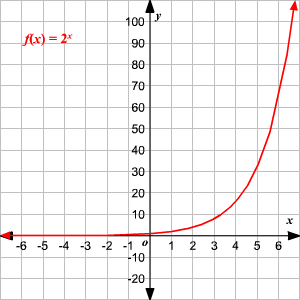If we replace   $x$ with $-x$   to get the equation $g\left(x\right)={2}^{-x}$ ,  the graph gets reflected around the  $y$ -axis, but the domain and range do not change: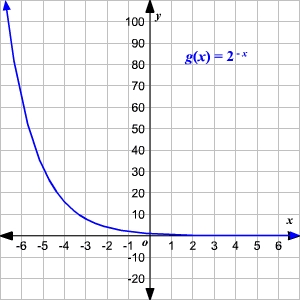If we put a negative sign in frontto get the equation $h\left(x\right)=-{2}^{x}$ , the graph gets reflected around the  $x$ -axis. We still have the whole real line as our domain, but the range is now the negative numbers, $y<0$ .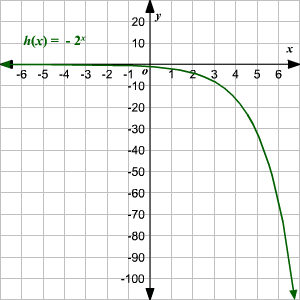Now, consider the function $f\left(x\right)={\left(-2\right)}^{x}$ . When $x=\frac{1}{2}$ , $y$ must be a complex number, so things get tricky. For this lesson we will require that our bases be positive for the moment, so that we can stay in the real-valued world.

In general, the graph of the basic exponential function $y={a}^{x}$ drops from $\infty$ to $0$  when $0  as $x$ varies from $-\infty$  to $\infty$ and rises from $0$ to $\infty$ when $a>1$ .

The exponential function $y={a}^{x}$ , can be shifted  $k$  units vertically and  $h$  units horizontally with the equation $y={a}^{\left(x+h\right)}+k$ . Then the domain of the function remains unchanged and the range becomes $\left\{y\in ℝ|y>h\right\}$ .

Example 1:

Find the domain and range of the function $y={3}^{x+2}$ .

Graph the function on a coordinate plane.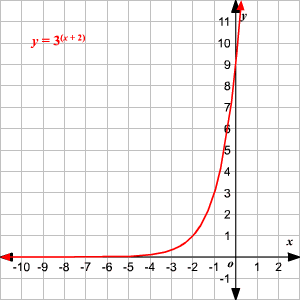The graph is nothing but the graph $y={3}^{x}$   translated $2$ units to the left.

The function is defined for all real numbers. So, the domain of the function is set of real numbers.

As $x$ tends to $\infty$ , the value of the function also tends to $\infty$   and as $x$ tends to $-\infty$ , the function approaches the $x$ -axis but never touches it.

Therefore, the range of the function is set of real positive numbers or $\left\{x\in ℝ|x>0\right\}$ .

Example 2:

Find the domain and range of the function $y={\left(\frac{1}{4}\right)}^{2x}$ .

Graph the function on a coordinate plane.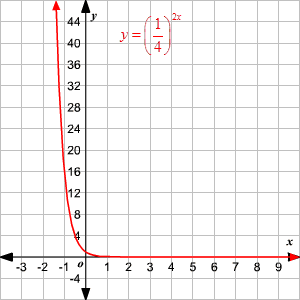The graph is nothing but the graph $y={\left(\frac{1}{4}\right)}^{x}$ compressed by a factor of $2$ .

The function is defined for all real numbers. So, the domain of the function is set of real numbers.

As $x$ tends to $\infty$ , the value of the function tends to zero and the graph approaches $x$ -axis but never touches it.

As $x$ tends to $-\infty$ , the function also tends to $\infty$ .

Therefore, the range of the function is set of real positive numbers or $\left\{y\in ℝ|y>0\right\}$ .

The inverse of an exponential function is a logarithmic function.

A simple logarithmic function $y={\mathrm{log}}_{2}x$  where $x>0$ is equivalent to the function $x={2}^{y}$ . That is, $y={\mathrm{log}}_{2}x$ is the inverse of the function $y={2}^{x}$ .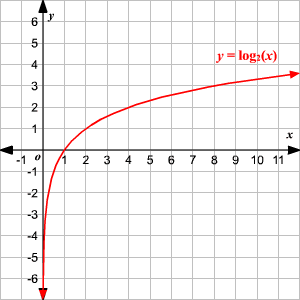The function $y={\mathrm{log}}_{2}x$ has the domain of set of positive real numbers and the range of set of real numbers.

Remember that since the logarithmic function is the inverse of the exponential function, the domain of logarithmic function is the range of exponential function, and vice versa.

In general, the function $y={\mathrm{log}}_{b}x$ where $b,x>0$ and $b\ne 1$ is a continuous and one-to-one function. Note that the logarithmic functionis not defined for negative numbers or for zero. The graph of the function approaches the $y$ -axis as $x$ tends to $\infty$ , but never touches it.

Therefore, the domain of the logarithmic function $y={\mathrm{log}}_{b}x$ is the set of positive real numbers and the range is the set of real numbers.

The function rises from $-\infty$ to $\infty$ as $x$ increases if $b>1$ and falls from $\infty$ to $-\infty$ as $x$ increases if $0 .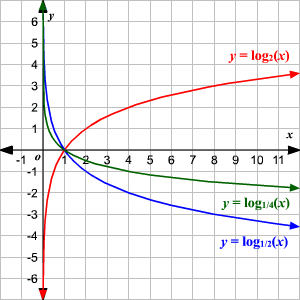The logarithmic function, $y={\mathrm{log}}_{b}x$ , can be shifted  $k$  units vertically and  $h$  units horizontally with the equation $y={\mathrm{log}}_{b}\left(x+h\right)+k$ . Then the domain of the function becomes $\left\{x\in ℝ|x>-h\right\}$ . However, the range remains the same.

Example 3:

Find the domain and range of the function $y=\mathrm{log}\left(x\right)-3$ .

Graph the function on a coordinate plane.Remember that when no base is shown, the base is understood to be $10$ .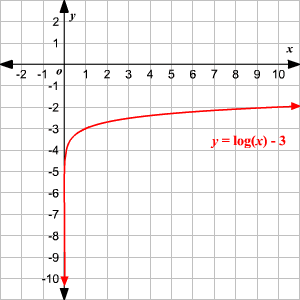The graph is nothing but the graph $y=\mathrm{log}\left(x\right)$ translated $3$ units down.

The function is defined for only positive real numbers. So, the domain of the function is set of positive real numbers or $\left\{x\in ℝ|x>0\right\}$ .

The function takes all the real values from $-\infty$ to $\infty$ .

Therefore, the range of the function is set of real numbers.

Example 4:

Find the domain and range of the function $y={\mathrm{log}}_{3}\left(x-2\right)+4$ .

Graph the function on a coordinate plane.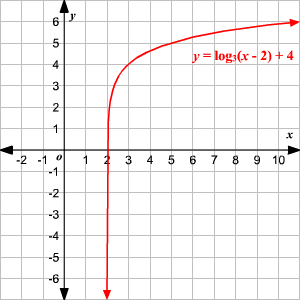The graph is nothing but the graph $y={\mathrm{log}}_{3}\left(x\right)$ translated $2$ units to the right and $4$ units up.

As $x$ tends to $2$ , the function approaches the line $x=2$ but never touches it. As $x$ tends to $\infty$ the value of the function also tends to $\infty$ . That is, the function is defined for real numbers greater than $2$ . So, the domain of the function is set of positive real numbers or $\left\{x\in ℝ|x>2\right\}$ .

The function takes all the real values from $-\infty$ to $\infty$ .

Therefore, the range of the function is set of real numbers.